Mean (or average) and median are statistical terms that have a somewhat similar role in terms of understanding the central tendency of a set of statistical scores. While an average has traditionally been a popular measure of a mid-point in a sample, it has the disadvantage of being affected by any single value being too high or too low compared to the rest of the sample. This is why a median is sometimes taken as a better measure of a mid point.

Comparison chart

Mean versus Median comparison chart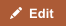MeanMedian
Definition The mean is the arithmetic average of a set of numbers, or distribution. It is the most commonly used measure of central tendency of a set of numbers. The median is described as the numeric value separating the higher half of a sample, a population, or a probability distribution, from the lower half.
Applicability The mean is used for normal distributions. The median is generally used for skewed distributions.
Relevance to the data set The mean is not a robust tool since it is largely influenced by outliers. The median is better suited for skewed distributions to derive at central tendency since it is much more robust and sensible.
How to calculate A mean is computed by adding up all the values and dividing that score by the number of values. The Median is the number found at the exact middle of the set of values. A median can be computed by listing all numbers in ascending order and then locating the number in the centre of that distribution.

Definitions of mean and median

In mathematics and statistics, the mean or the arithmetic mean of a list of numbers is the sum of the entire list divided by the number of items in the list. When looking at symmetric distributions, the mean is probably the best measure to arrive at central tendency. In probability theory and statistics, a median is that number separating the higher half of a sample, a population, or a probability distribution, from the lower half.

How to calculate

The Mean or average is probably the most commonly used method of describing central tendency. A mean is computed by adding up all the values and dividing that score by the number of values. The arithmetic mean of a sample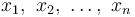$x_1,\ x_2,\ \ldots,\ x_n$ is the sum the sampled values divided by the number of items in the sample: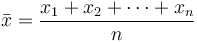$\bar{x} = \frac{x_1+x_2+\cdots +x_n}{n}$

The Median is the number found at the exact middle of the set of values. A median can be computed by listing all numbers in ascending order and then locating the number in the center of that distribution. This is applicable to an odd number list; in case of an even number of observations, there is no single middle value, so it is a usual practice to take the mean of the two middle values.

Example

Let us say that there are nine students in a class with the following scores on a test: 2, 4, 5, 7, 8, 10, 12, 13, 83. In this case the average score (or the mean) is the sum of all the scores divided by nine. This works out to 144/9 = 16. Note that even though 16 is the arithmetic average, it is distorted by the unusually high score of 83 compared to other scores. Almost all of the students' scores are below the average. Therefore, in this case the mean is not a good representative of the central tendency of this sample.

The median, on the other hand, is the value which is such that half the scores are above it and half the scores below. So in this example, the median is 8. There are four scores below and four above the value 8. So 8 represents the mid point or the central tendency of the sample.

Disadvantages of Arithmetic Means and Medians

Mean is not a robust statistic tool since it cannot be applied to all distributions but is easily the most widely used statistic tool to derive the central tendency. The reason that mean cannot be applied to all distributions is because it gets unduly impacted by values in the sample that are too small to too large.

The disadvantage of median is that it is difficult to handle theoretically. There is no easy mathematical formula to calculate the median.

Other Types of Means

There are many ways to determine the central tendency, or average, of a set of values. The mean discussed above is technically the arithmetic mean, and is the most commonly used statistic for average. There are other types of means:

Geometric Mean

The geometric mean is defined as the nth root of the product of n numbers, i.e., for a set of numbers x1,x2,...,xn, the geometric mean is defined as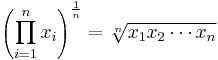$\left(\prod_{i=1}^n x_i\right)^\frac{1}{n} = \sqrt[n]{x_1 x_2 \cdots x_n}$

Geometric means are better than arithmetic means for describing proportional growth. For example, a good application for geometric mean is calculating the compounded annual growth rate (CAGR).

Harmonic Mean

The harmonic mean is the reciprocal of the arithmetic mean of the reciprocals. The harmonic mean H of the positive real numbers x1,x2,...,xn is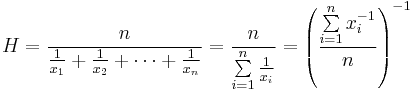$H = \frac{n}{\frac1{x_1} + \frac1{x_2} + \cdots + \frac1{x_n}} = \frac{n}{\sum\limits_{i=1}^n \frac1{x_i}} = \left(\frac{\sum\limits_{i=1}^n x_i^{-1}}{n}\right)^{-1}$

A good application for harmonic means is when averaging multiples. For exampe, it is better to use weighted harmonic mean when calculating the average price–earnings ratio (P/E). If P/E ratios are averaged using a weighted arithmetic mean, high data points get unduly greater weights than low data points.

Pythagorean Means

The arithmetic mean, geometric mean and harmonic mean together form a set of means called the Pythagorean means. For any set of numbers, the harmonic mean is always the smallest of all Pythagorean means, and the arithmetic mean is always the largest of the 3 means. i.e. Harmonic mean ≤ Geometric mean ≤ Arithmetic mean.

Other meanings of the words

Mean can be used as a figure of speech and holds a literary reference. It is also used to imply poor or not being great. Median, in a geometric reference, is a straight line passing from a point in the triangle to the centre of the opposite side.